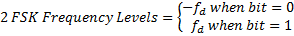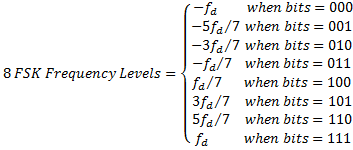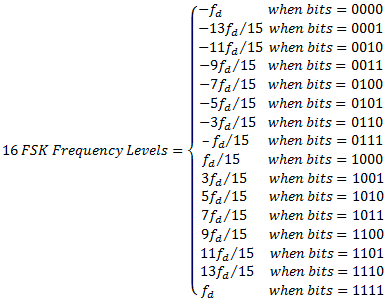# Frequency-Shift Keying (FSK)

NI-RFmx Demod 3.0 Help

Edition Date: January 2019

Part Number: 374641K-01

»View Product InfoDownload Help (Windows Only)

FSK refers to a type of frequency modulation that assigns bit values to discrete frequency levels. The supported M-ary values for FSK are 2, 4, 8, and 16.

The FSK demodulator uses the discrete levels in frequency as the symbol map. The recovered frequency symbol closest to the ideal symbol in the map decides the bits it represents. The bits mentioned in the symbol map are read as b(log2M)-1 ... b0.

The bit stream returned after analyzing the complete waveform is reported as follows:

{b0 ... b(log2M)-1}0 {b0 ... b(log2M)-1}1 ... {b0 ... b(log2M)-1}n-1

where M is the M-ary and n is the number of symbols analyzed.

Let fd be the frequency deviation of the modulated signal. Then, for a specified M-ary, the frequency levels are defined as follows:

fi = -fd + 2ifd/(M-1)

where 0 >= i < = M-1

The default symbol map is as shown in the following equations: